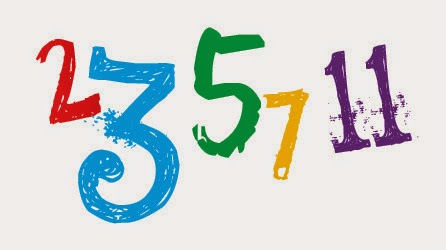## Find a Number is Prime or not in C Language with Example

Find a Number is Prime or not in C Language with Example.
C program to find a Number is prime or not. It is a simple source code that provides the output of an actual Prime number. Take care of a questions for your interviews or students exam. This is a totally free of cost code.NUMBER IS PRIME OR NOT IN C
This C program is used find the given number is prime or not by using different statements like looping & unconditional statement. Check the entered value with condition of if(p_flag == 1). If it is satisfies the condition, then print a number as prime.  Otherwise it is not a prime number.

Q: Write a C program to check whether a given input value is Prime number or not.

//Header files
#include<stdio.h>
#include<conio.h>
//Global variable
int isPrime(int);

void main()
{
//Program variables
int cr,num,p_flag=0;

clrscr(); //Function to clear previous output
printf("Enter the value:"); //Display function
scanf("%d",&num); //Getting input value function
}
int isPrime(num)
{
for(cr=2;cr<num/2;cr++) //Looping statement
{
if(num%cr==0//Conditional statement
{
p_flag=1;
break;
}
}
}

if(p_flag == 1)
printf("Number is not prime");
else
printf("Number is prime");
getch();
}

#### Post a comment

Note: only a member of this blog may post a comment.

## Labels

Designed By Blogger Templates